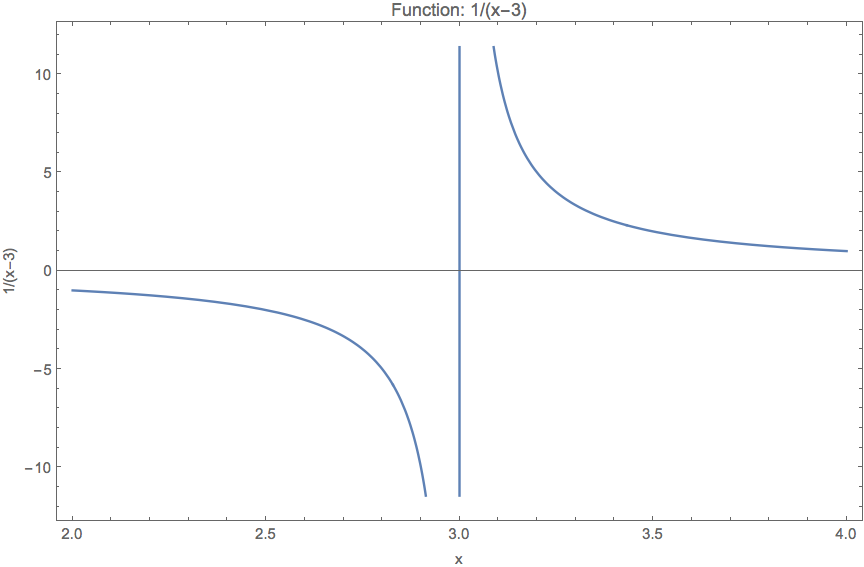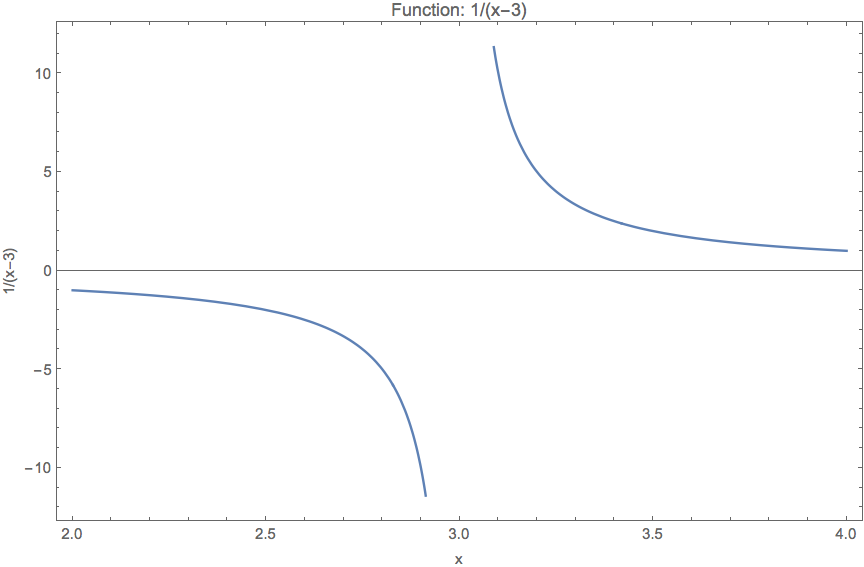Mathematica Plot might include some non-existant lines sometimes, Exclusions is the potion for it.

Mathematica Plot function brings in weird lines sometimes.

Here is an example of it. I plot

$$f(x) = \frac{1}{1-0.3 x}.$$

Plot[1/(1 - 0.3 x), {x, 2, 5}, Frame -> True,
FrameLabel -> {"x", "1/(1-0.3 x)"}, ImageSize -> Large,
PlotLabel -> "Function: 1/(1-0.3 x)"]


What we get is a plotA plot with singularity point. Mathematica Plot brings in an extra line at the singularity.

This vertical line at $x=3$ is not real. To eliminate this line, we can exclude the singularity point when plotting, using Exclusions.

Plot[1/(x - 3), {x, 2, 4}, Frame -> True,
FrameLabel -> {"x", "1/(x-3)"}, ImageSize -> Large,
PlotLabel -> "Function: 1/(x-3)", Exclusions -> {3}]


The plot we get now is much better.With Exclusions->{3}

Published: by ;

Lei Ma (2017). 'Mathematica Exclude Singularities in Plot', Datumorphism, 03 April. Available at: https://datumorphism.leima.is/til/programming/mathematica/mathematica-plot-exclude-singularities/.

Table of Contents

Current Ref:

• til/programming/mathematica/mathematica-plot-exclude-singularities.md